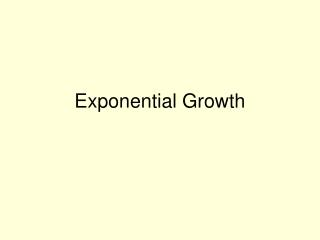# Exponential Growth - PowerPoint PPT PresentationDownload PresentationExponential Growth

Exponential GrowthDownload Presentation## Exponential Growth

- - - - - - - - - - - - - - - - - - - - - - - - - - - E N D - - - - - - - - - - - - - - - - - - - - - - - - - - -
##### Presentation Transcript

1. Exponential Growth

2. Exponential Growthy = a (1 +b)t y = final amount after a period of timea= original amountt = timeb = percent of increase (as a decimal)(1 + b ) is called the multiplierIf multiplier is greater than 1, it is a growth problem

3. Finding the multiplierex. Growth of 5%Change 5% to a decimal (.05)1+ .05 = 1.051.05 is the multiplier

4. Ex. A certain quantity DoublesIf sometime doubles, it is increasing by 100%100% = 1.01+ 1.0 = 2 Therefore, 2 is the multiplier

5. A quantity triplesIf something triples it is increasing by 200% 200% = 2.01 + 2.0 = 33 is the multiplier

6. Finding percent of growthPercent of growth= amount of changeoriginal amountMake this decimal a %This is the % of change

7. Ex. A quantity changes from 20 to 35. Find the percent of increase.Amount of change = 15 Orignal Amount = 20 = .75 = 75%75% is the percent of increase

8. Ex. A price increases from \$40 to \$45. Find the % of increase 5 = 1 40 8= .125 = 12.5%

9. Ex. The population of a town increases from 60,000 to 120,000. Find the % of increase.60,00060,000= 1.0 = 100%100% is the percent of increase

10. Examples of Exponential Growth

11. Ex. The price of a gallon of milk increases at a rate of 2% per year. In 1990, a gallon of milk cost \$2. How much would the gallon of milk be expected to cost in 2002?Final cost=original amt (1 +% ofincrease)time cost in 2002 = 2(1+.02)12 = 2(1.02)12 = \$2.54

12. Ex. The population of a town is increasing at a rate of 6% every 2 years. The population was 12,000 in 1980. What would the population be expected to be in 2000?Population in 2000 = 12,000(1 + .06)10 = 12,000(1.06)10 21,490

13. The # of deer in a particular forest is estimated to be about 352 right now. The population has been growing at a rate of 12% What was the approximate population 5 years ago?

14. The value of an investment has grown at a rate of 2% per year It was originally valued at \$20,000. It is now valued at \$22,974. How much time has gone by?

15. 22,974 = 20,000(1.02)t 22,974 = 20,000(1.02)t 20,000 = 20,0001.15 ≈ (1.02)t *Next use “guess and check” for values of “t”(1.02)5 ≈ 1.10(1.02)6 ≈ 1.13(1.02)7 ≈ 1.15This last one is closest to 1.15 so 7 years have gone by.